﻿ Does Temperature Effects the Growth of Microcracks in a Casted Broken Femur?Publications are Open
Access in this journal
Article Versions
Export Article
• Normal Style
• MLA Style
• APA Style
• Chicago Style
Research Article
Open Access Peer-reviewed

### Does Temperature Effects the Growth of Microcracks in a Casted Broken Femur?

Mary Tsili , D. Zacharopoulos
Journal of Biomedical Engineering and Technology. 2017, 5(1), 20-24. DOI: 10.12691/jbet-5-1-4
Published online: July 19, 2017

### Abstract

We considered if temperature effects the growth of microcracks in a casted bro- ken femur, locally at three particularly points. We used theory of adaptive elasticity neglecting and accounting temperature and energy density theory. We showed for both cases after the removal of the cast, femur locally at points of our interest i) will be weaken (the mean length of their microcracks will be increased) or ii) will be under osteoporosis (the mean length of their microcracks will be dramatically increased). The results coincide with those of corresponding problem at macroscopic area. We resulted that temperature plays no role to growth of microcracks for our case.

### 1. Introduction

The purpose of this paper is to study if temperature effects the growth of microcracks in a broken castedfemur. For that reason we will use the theory of internal bone remodeling 1, 2 at microscopic area neglecting and accounting temperature and the energy density theory 3, 4, 5.

Macroscopically the bone has a volume V and a surface S. The volume V microscopically consists of microvolumes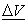which generally are not homogenous 3. Expanding the theory of adaptive elasticity [ 1, p. 322] at microscopic area we assume:

i) Every micro volumeof bone consists of a elastic microvolume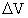(micromatrix bone) and of microporous (microcracks) that is: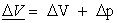(1)

where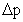is the volume of microcracks.

From the other hand Sih [ 5, p.179] showed that every microvolumecontains an homogenous microvolume. Thus we suppose that an elastic microvolumegiven by (1) is homogenous microvolume.

ii) The mechanical properties of microvolume of bonecoincides with the mechanical properties of homogenous microvolumeof micromatrix bone.

iii)The fraction of microvolume of the micromatrix bone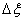is defined as [ 1, p. 322]: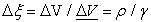(2)

where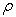is the density of microvolume, while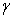is the density of material (bone) and assume to be cons- tant. From the above it follows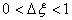.

v) The porosity that is the mean length of microcraks of microvolumealters with mass added /removal to /from micro matrix bone and linearly depends from the history of microstrain [ 1, p. 322]. The above is characterized by a parameter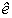2: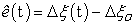(3)

where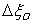is the initial fraction of the microvolume of of micromatrix bone. With other words parameteris the change of the mean value of microcracks.

### 2. The Problem and Its Physical Approximation

Assume that someone with bones that are not un- der osteoporosis 2 breaks his /her femur. We cast bone for a period 6-8 weeks. In earliest paper we studied same problem at macroscopic area 6. In present paper we want to predict the situation of healing bone after the removal of cast, locally to three points indicated in Figure 1:

A: at the top of inner rad of femur’s diaphysis

B: at the middle of outer rad of femur’s diaphysis and

Γ: at the bottom of middle distance between inner and outer rad of femur’s diaphysis.

Αssume that at t= 0 broken femur at macroscopic area was under a constant tensile load Τo, due to the total weight of the leg (femur + tibia). Consequently its points Α, Β and Γ were respectively under constant tensile loads Go, Fo and Ρο. The lasts due respectively to the total weights: i) of leg (femur + tibia), ii)of half fe- mur +tibia and iii) of tibia. All loads are in units of B.W. Finally Α, Β andhad the sameand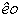2.

View option
• Figure 1. A cylinder length L with inner and outer radii a and b respectively and points: Α Β, Γ. The area ΔΕ is rupture

At t >0 we cast broken femur for a period of 6-8 weeks. Then the femur locally at points Α, Β and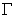continues to be under the same tensile loads Go, Fo and Ρο respectively since patient can not walk and uses crosiers. In addition points Β andare under a con- stant external pressure P1 due to cast. We want to predict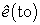, where to is time moment of removal of cast.

### 3. A Hollow Circular Cylinder Subjected to an External Pressure and to Axial Load

Femur is modeled as a hollow circular cylinder with length L, inner and outer radii a and b respectively indicated in Figure 1. These radii correspond to endosteal and periosteal surface of bone and are constant, due to internal remodeling 7. Since we deal with microscopic area, we base upon density energy theory 3, 4, 5.

The equations of above theory in cylindrical coordinates are the followings:

i) the stress relations between macroscopic and microscopic area [ 3, p.182]where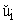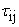,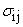and (dV/dA) are respectively: the macroscopic displacement, the stress on microvolume, the stress at macrovolume and the change of volume with surface 3.

ii) the macrostress - equations [ 5, p.182]: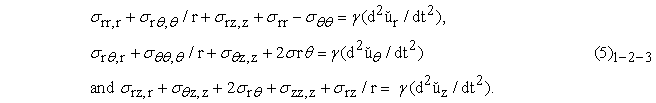iii) the microstress equations [ 5, p.182]: :where ú denotes microdisplacement.

iv) the microstrain-microdisplacement relations [ 5, p.179]:v) the microstress - microstrain relations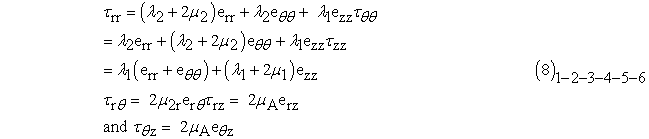where: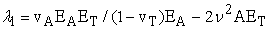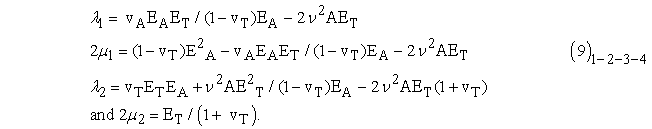In the above ΕΑ, ΕΤ and vA,, vΤ are Young’s modulus and Poisson ratio in transverse and axial direction in macroscopic area.

Finally rate remodeling equation 2 microscopic area without /and accounting temperature are respectively: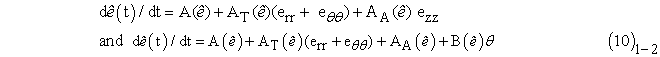where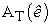,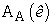are rate remodeling coefficients in transverse and axial direction respectively, while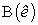is rate remodeling coefficient depends from temperature.

The boundary conditions of our problem are:

i) at point A: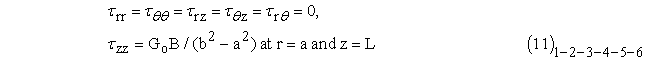ii) at point B: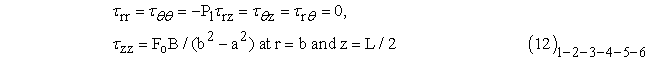iii) at point: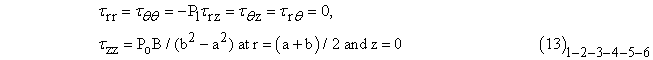where B is the body weight of the pattient and suppose to be constant during casting.

Our problem has a unique solution [ 5, p.186] and assume that microdisplacements are of the form: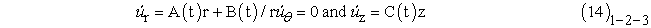where A(t), B(t) and C(t) are unknowns. Then (7) are written as:Therefore (8) because of (15) take the forms: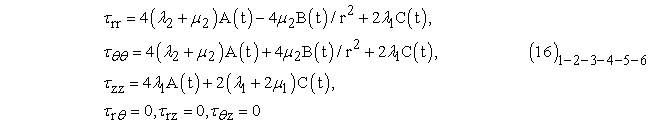Applying the boundary conditions (11)–(12)−(13) into (16) it is possible to obtain A(t), B(t), C(t) at points Α, Β and Γ respectively. Thus:

i) at point Α:ii) at point Β: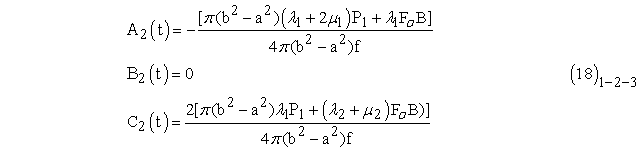iii) at point: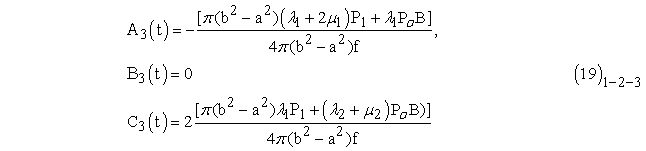where: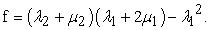(20)

Employing (17), (18) and (19) into (14), it possible to obtain the microdisplacements at points Α, Β and Γ respectively. Also employing (17),(18) and (19) into (15), it is possible to obtain microstrains. Thus:

i) at point Α:ii) at point Β:iii) at point:At continuity we distinguish the following cases:

i) The internal remodeling of femur does not depends upon temperature:

Then substituting (21), (22), (23) into (10)1 it is possible to obtain:

i) at point Α: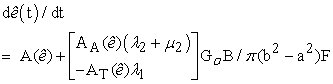(24)

ii) at point Β: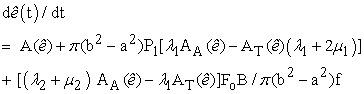(25)

iii) at point: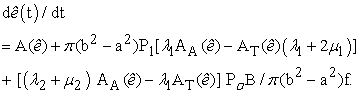(26)

Since living bone is continually remodeling obeying to Wollf’s law 8, 9 we assume Young’s modulus and Poisson’s ratio depends upon το6, 10, 11, 12. Then from (6) it results that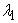,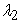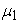,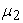depend also. At continuity we impose:Therefore(24)-(25)-(26) conclude to the following form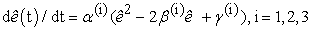(28)

where:

i) at point Α:ii) at point Β: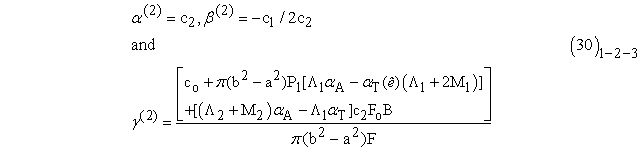iii) at point: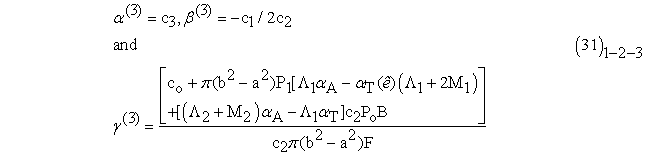where: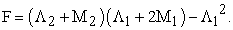(32)

As we stated earlier initially femur was in a state at which no remodeling occured. Therefore 2: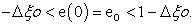(33)

The solutions of (28) satisfying (33) are:The acceptable solutions are given in Table 1. 6. Αccordingly to results of this Table there are two possible cases: After the removal of cast the femur at points A, B, Γ: i) will be weaken, that is the mean length of microcraks will be increased or ii) will be under osteoporosis, that is the mean length of microcraks will be dramatically increased.

ii) The internal remodeling of femur does depends upon temperature:

Then substituting (21),(22),(23) into (10)2 it is possible to obtain:

i) at point Α: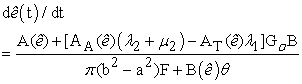(35)

ii) at point Β: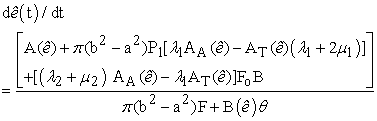(36)

iii) at point: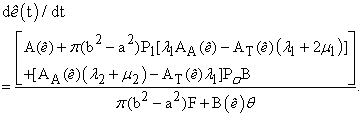(37)

At continuity substituting (27) into (35), (36), (37) and imposing: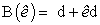(38)

we again conclude to (28) where:

i) at point Α:ii) at point Β: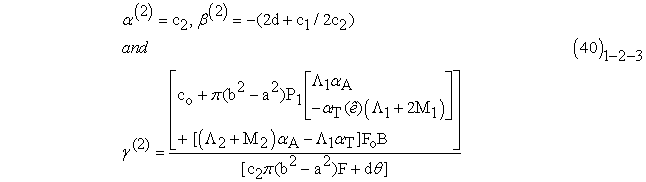iii) at point: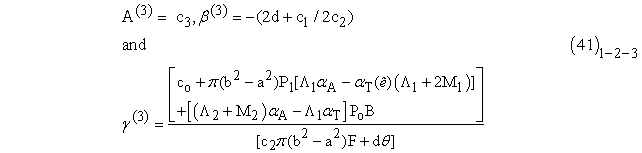The solutions of (28) satisfying (33) are (34)1-2 and all that we stated at previous case are valid.

### 4. Discussion and Conclusion

Our results coincides with the corresponding problem at macroscopic area 6, 13, 14. Therefore both accounting and neglecting temperature after the removal of cast the femur locally at points of our interest: will be weaken or osteoporotic, that is an increase or a dramatically increase of its microcracks will be arised. From the above we conclude that temperature does not effect the growth of micro- cracks in a broken casted femur.

### References

  Cowin S. and Hegedus D. (1976). Bone remode-ling I: Theory of adaptive elasticity”. J. Elastic. 6, pp. 313-326. In article View Article  Hegedus D. και Cowin S. (1976). Bone remodeling II: Theory of adaptive elasticity”. J. Elastic. 6, pp. 337-352. In article View Article  Sih G.C. (1985). “Mechanics and Physics of energy density theory”, Theoret., Appl., Fract. , Mech., 44, pp. 157-173. In article View Article  Sih G.C (1972 -1982). “Mechanics of fracture, Introductory chapters”, Vol. I- VII, edited by G.C. Sih, Marti-nus Nijhoff, The Hague. In article  Sih GC (1988). “Thermomechanics of solids: none-quilibrium and irreversibility”, Theoretical and Applied Fracture Mechanics, 9, pp. 175-198. In article View Article  Tsili M. (2000). “Theoretical solutions for internal bone remodeling of diaphyseal shafts using adaptive elasticity theory” J. Biomech., 33 pp. 235-239. In article View Article  Frost H. M. (1964). “Dynamics of bone remodeling in bone bio dynamics” (edited by Frost H.M) Little and Brown 316, Boston. In article PubMed  Wolff. J. (1884). Das gesetz der transformation der inneren architecture knocken bei pathologism veran-derungen der aussen knochenform. Sitz Ber. Preuss Acad. d. Wiss 22, Sitz Physik- Math. K1. In article  Wolff J. (1892). “Das gesetz der transformation knocken hirschald”, Berlin In article  Cowin S. and Van-Burskirk W. (1978). “Internal bone remodeling induced by a medullary pin.” J. Biomech. 11, pp. 269-275. In article View Article  Τsili M. (2008b). “Internal bone remodeling induced by the distance - running and the unkown remodeling coefficients” in: www.ispub.com/journal- of- internet journal of bioengineering, Volume 4. number 2, In article  Τsili M. (2008c). “Internal bone remodeling induced by volleyball in: www.ispub.com/journal-of-internet journal of bioengineering, Volume. 4. number 1. In article  Dietrick J., Whedon, Shore E. (1948) American Journal of Medicine 4, pp. 3-36. In article View Article  Fung Y. (1981). Biomechanics. Mechanical proper- ties of living tissues, springer, New York. In article View ArticleThis work is licensed under a Creative Commons Attribution 4.0 International License. To view a copy of this license, visit http://creativecommons.org/licenses/by/4.0/